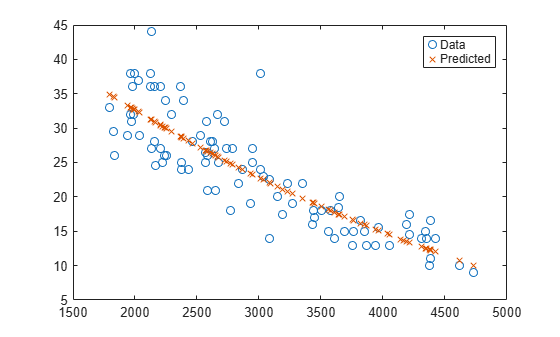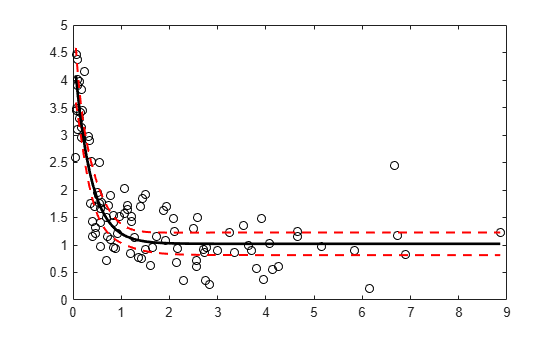# predict

Predict response of nonlinear regression model

## Syntax

``ypred = predict(mdl,Xnew)``
``````[ypred,yci] = predict(mdl,Xnew)``````
``````[ypred,yci] = predict(mdl,Xnew,Name,Value)``````

## Description

example

````ypred = predict(mdl,Xnew)` returns the predicted response of the nonlinear regression model `mdl` to the points in `Xnew`.```

example

``````[ypred,yci] = predict(mdl,Xnew)``` also returns confidence intervals for the responses at `Xnew`.```

example

``````[ypred,yci] = predict(mdl,Xnew,Name,Value)``` specifies additional options using one or more name-value arguments. For example, you can specify the confidence level of the confidence interval and the prediction type.```

## Examples

collapse all

Create a nonlinear model of car mileage as a function of weight, and predict the response.

Create an exponential model of car mileage as a function of weight from the `carsmall` data. Scale the weight by a factor of 1000 so all the variables are roughly equal in size.

```load carsmall X = Weight; y = MPG; modelfun = 'y ~ b1 + b2*exp(-b3*x/1000)'; beta0 = [1 1 1]; mdl = fitnlm(X,y,modelfun,beta0);```

Create predicted responses to the data.

```Xnew = X; ypred = predict(mdl,Xnew);```

Plot the original responses and the predicted responses to see how they differ.

```plot(X,y,'o',X,ypred,'x') legend('Data','Predicted')```Create a nonlinear model of car mileage as a function of weight, and examine confidence intervals of some responses.

Create an exponential model of car mileage as a function of weight from the `carsmall` data. Scale the weight by a factor of 1000 so all the variables are roughly equal in size.

```load carsmall X = Weight; y = MPG; modelfun = 'y ~ b1 + b2*exp(-b3*x/1000)'; beta0 = [1 1 1]; mdl = fitnlm(X,y,modelfun,beta0);```

Create predicted responses to the smallest, mean, and largest data points.

```Xnew = [min(X);mean(X);max(X)]; [ypred,yci] = predict(mdl,Xnew)```
```ypred = 3×1 34.9469 22.6868 10.0617 ```
```yci = 3×2 32.5212 37.3726 21.4061 23.9674 7.0148 13.1086 ```

Generate sample data from the nonlinear regression model

`$y={b}_{1}+{b}_{2}\mathrm{exp}\left(-{b}_{3}x\right)+ϵ$`

where ${b}_{1}$, ${b}_{2}$, and ${b}_{3}$ are coefficients, and the error term $ϵ$ is normally distributed with mean 0 and standard deviation 0.5.

```modelfun = @(b,x)(b(1)+b(2)*exp(-b(3)*x)); rng('default') % For reproducibility b = [1;3;2]; x = exprnd(2,100,1); y = modelfun(b,x) + normrnd(0,0.5,100,1);```

Fit the nonlinear model using robust fitting options.

```opts = statset('nlinfit'); opts.RobustWgtFun = 'bisquare'; b0 = [2;2;2]; mdl = fitnlm(x,y,modelfun,b0,'Options',opts);```

Plot the fitted regression model and simultaneous 95% confidence bounds.

```xrange = [min(x):.01:max(x)]'; [ypred,yci] = predict(mdl,xrange,'Simultaneous',true); figure() plot(x,y,'ko') % observed data hold on plot(xrange,ypred,'k','LineWidth',2) plot(xrange,yci','r--','LineWidth',1.5)``````S = load('reaction'); X = S.reactants; y = S.rate; beta0 = S.beta;```

Specify a function handle for observation weights, then fit the Hougen-Watson model to the rate data using the specified observation weights function.

```a = 1; b = 1; weights = @(yhat) 1./((a + b*abs(yhat)).^2); mdl = fitnlm(X,y,@hougen,beta0,'Weights',weights);```

Compute the 95% prediction interval for a new observation with reactant levels [100,100,100] using the observation weight function.

```[ypred,yci] = predict(mdl,[100,100,100],'Prediction','observation', ... 'Weights',weights)```
```ypred = 1.8149 ```
```yci = 1×2 1.5264 2.1033 ```

## Input Arguments

collapse all

Nonlinear regression model object, specified as a `NonLinearModel` object created by using `fitnlm`.

New predictor input values, specified as a table, dataset array, or matrix. Each row of `Xnew` corresponds to one observation, and each column corresponds to one variable.

• If `Xnew` is a table or dataset array, it must contain predictors that have the same predictor names as in the `PredictorNames` property of `mdl`.

• If `Xnew` is a matrix, it must have the same number of variables (columns) in the same order as the predictor input used to create `mdl`. Note that `Xnew` must also contain any predictor variables that are not used as predictors in the fitted model. Also, all variables used in creating `mdl` must be numeric. To treat numerical predictors as categorical, identify the predictors using the `'CategoricalVars'` name-value pair argument when you create `mdl`.

Data Types: `single` | `double` | `table`

### Name-Value Arguments

Specify optional pairs of arguments as `Name1=Value1,...,NameN=ValueN`, where `Name` is the argument name and `Value` is the corresponding value. Name-value arguments must appear after other arguments, but the order of the pairs does not matter.

Before R2021a, use commas to separate each name and value, and enclose `Name` in quotes.

Example: ```[ypred,yci] = predict(Mdl,Xnew,'Alpha',0.01,'Simultaneous',true)``` returns the confidence interval `yci` with a 99% confidence level, computed simultaneously for all predictor values.

Significance level for the confidence interval, specified as the comma-separated pair consisting of `'Alpha'` and a numeric value in the range [0,1]. The confidence level of `yci` is equal to 100(1 – `Alpha`)%. `Alpha` is the probability that the confidence interval does not contain the true value.

Example: `'Alpha',0.01`

Data Types: `single` | `double`

Prediction type, specified as the comma-separated pair consisting of `'Prediction'` and either `'curve'` or `'observation'`.

A regression model for the predictor variables X and the response variable y has the form

y = f(X) + ε,

where f is a fitted regression function and ε is a random noise term.

• If `'Prediction'` is `'curve'`, then `predict` predicts confidence bounds for f(Xnew), the fitted responses at `Xnew`.

• If `'Prediction'` is `'observation'`, then `predict` predicts confidence bounds for y, the response observations at `Xnew`.

The bounds for y are wider than the bounds for f(X) because of the additional variability of the noise term.

Example: `'Prediction','observation'`

Flag to compute simultaneous confidence bounds, specified as the comma-separated pair consisting of `'Simultaneous'` and either true or false.

• `true``predict` computes confidence bounds for the curve of response values corresponding to all predictor values in `Xnew`, using Scheffe's method. The range between the upper and lower bounds contains the curve consisting of true response values with 100(1 – α)% confidence.

• `false``predict` computes confidence bounds for the response value at each observation in `Xnew`. The confidence interval for a response value at a specific predictor value contains the true response value with 100(1 – α)% confidence.

Simultaneous bounds are wider than separate bounds, because requiring the entire curve of response values to be within the bounds is stricter than requiring the response value at a single predictor value to be within the bounds.

Example: `'Simultaneous',true`

Vector of real, positive value weights or a function handle.

• If you specify a vector, then it must have the same number of elements as the number of observations (or rows) in `Xnew`.

• If you specify a function handle, the function must accept a vector of predicted response values as input, and returns a vector of real positive weights as output.

Given weights, `W`, `predict` estimates the error variance at observation `i` by `MSE*(1/W(i))`, where MSE is the mean squared error.

## Output Arguments

collapse all

Predicted response values evaluated at `Xnew`, returned as a numeric vector.

Confidence intervals for the responses, returned as a two-column matrix with each row providing one interval. The meaning of the confidence interval depends on the settings of the name-value pair arguments `'Alpha'`, `'Prediction'`, and `'Simultaneous'`.

## Tips

 Lane, T. P. and W. H. DuMouchel. “Simultaneous Confidence Intervals in Multiple Regression.” The American Statistician. Vol. 48, No. 4, 1994, pp. 315–321. Available at https://doi.org/10.1080/00031305.1994.10476090

 Seber, G. A. F., and C. J. Wild. Nonlinear Regression. Hoboken, NJ: Wiley-Interscience, 2003.